## Friday, May 7, 2010

### How froc works

I am happy to announce the release of version 0.2 of the `froc` library for functional reactive programming in OCaml. There are a number of improvements:

• better event model: there is now a notion of simultaneous events, and behaviors and events can now be freely mixed
• self-adjusting computation is now supported via memo functions; needless recomputation can be avoided in some cases
• faster priority queue and timeline data structures
• behavior and event types split into co- and contra-variant views for subtyping
• bug fixes and cleanup

Development of `froc` has moved from Google Code to Github; see

Thanks to Ruy Ley-Wild for helpful discussion, and to Daniel Bünzli for helpful discussion and many good ideas in React.

I thought I would take this opportunity to explain how `froc` works, because it is interesting, and to help putative `froc` users use it effectively.

Dependency graphs

The main idea behind `froc` (and self-adjusting computation) is that we can think of an expression as implying a dependency graph, where each subexpression depends on its subexpressions, and ultimately on some input values. When the input values change, we can recompute the expression incrementally by recursively pushing changes to dependent subexpressions.

To be concrete, suppose we have this expression:

``````  let u = v / w + x * y + z
``````

Here is a dependency graph relating expressions to their subexpressions: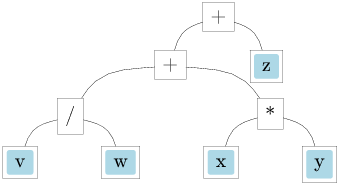The edges aren’t directed, because we can think of dependencies as either demand-driven (to compute A, we need B), or change-driven (when B changes, we must recompute A).

Now suppose we do an initial evaluation of the expression with ```v = 4```, `w = 2`, `x = 2`, `y = 3`, and `z = 1`. Then we have (giving labels to unlabelled nodes, and coloring the current value of each node green):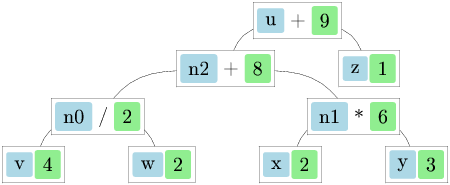If we set `z = 2`, we need only update `u` to `10`, since no other node depends on `z`. If we then set `v = 6`, we need to update `n0` to `3`, `n2` to `9` (since `n2` depends on `n0`), and `u` to `11`, but we don’t need to update `n1`. (This is the change-driven point of view.)

What if we set `z = 2` and `v = 6` simultaneously, then do the updates? We have to be careful to do them in the right order. If we updated `u` first (since it depends on `z`), we’d use a stale value for `n2`. We could require that we don’t update an expression until each of its dependencies has been updated (if necessary). Or we could respect the original evaluation order of the expressions, and say that we won’t update an expression until each expression that came before it has been updated.

In `froc` we take the second approach. Each expression is given a timestamp (not a wall-clock time, but an abstract ordered value) when it’s initially evaluated, and we re-evaluate the computation by running through a priority queue of stale expressions, ordered by timestamp. Here is the situation, with changed values in magenta, stale values in red, and timestamps in gray:If we update the stale nodes from their dependencies in timestamp order, we get the right answer. We will see how this approach gives us a way to handle control dependencies, where A does not depend on B, but A’s execution is controlled by B.

Library interface

The core of `froc` has the following (simplified) signature:

``````  type 'a t
val return : 'a -> 'a t
val bind : 'a t -> ('a -> 'b t) -> 'b t
``````

The type `'a t` represents changeable values (or just changeables) of type `'a`; these are the nodes of the dependency graph. `Return` converts a regular value to a changeable value. `Bind` makes a new changeable as a dependent of an existing one; the function argument is the expression that computes the value from its dependency. We have `>>=` as an infix synonym for `bind`; there are also multi-argument versions (`bind2`, `bind3`, etc.) so a value can depend on more than one other value.

We could translate the expression from the previous section as:

``````  let n0 = bind2 v w (fun v w -> return (v / w))
let n1 = bind2 x y (fun x y -> return (x * y))
let n2 = bind2 n0 n1 (fun n0 n1 -> return (n0 + n1))
let u = bind2 n2 z (fun n2 z -> return (n2 + z))
``````

There are some convenience functions in `froc` to make this more readable (these versions are also more efficient):

``````  val blift : 'a t -> ('a -> 'b) -> 'b t
val lift : ('a -> 'b) -> 'a t -> 'b t
``````

`Blift` is like `bind` except that you don’t need the `return` at the end of the expression (below we’ll see cases where you actually need `bind`); `lift` is the same as `blift` but with the arguments swapped for partial application. So we could say

``````  let n0 = blift2 v w (fun v w -> v / w)
let n1 = blift2 x y (fun x y -> x * y)
let n2 = blift2 n0 n1 (fun n0 n1 -> n0 + n1)
let u = blift2 n2 z (fun n2 z -> n2 + z)
``````

or even

``````  let (/) = lift2 (/)
let ( * ) = lift2 ( * )
let (+) = lift2 (+)
let u = v / w + x * y + z
``````

Now, there is no reason to break down expressions all the way—a node can have a more complicated expression, for example:

``````  let n0 = blift2 v w (fun v w -> v / w)
let n2 = blift3 n0 x y (fun n0 x y -> n0 + x * y)
let u = blift2 n2 z (fun n2 z -> n2 + z)
``````

There is time overhead in propagating dependencies, and space overhead in storing the dependency graph, so it’s useful to be able to control the granularity of recomputation by trading off computation over changeable values with computation over ordinary values.

Dynamic dependency graphs

Take this expression:

``````  let b = x = 0
let y = if b then 0 else 100 / x
``````

Here it is in `froc` form:

``````  let b = x >>= fun x -> return (x = 0)
let n0 = x >>= fun x -> return (100 / x)
let y = bind2 b n0 (fun b n0 -> if b then return 0 else n0)
``````

and its dependency graph, with timestamps: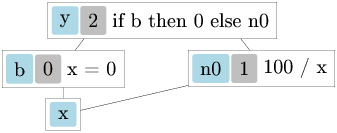(We begin to see why `bind` is sometimes necessary instead of `blift`—in order to return `n0` in the `else` branch, the function must return `'b t` rather than `'b`.)

Suppose we have an initial evaluation with `x = 10`, and we then set `x = 0`. If we blindly update `n0`, we get a `Division_by_zero` exception, although we get no such exception from the original code. Somehow we need to take into account the control dependency between `b` and `100 / x`, and compute `100 / x` only when `b` is false. This can be accomplished by putting it inside the `else` branch:

``````  let b = x >>= fun x -> return (x = 0)
let y = b >>= fun b -> if b then return 0
else x >>= fun x -> return (100 / x)
``````

How does this work? `Froc` keeps track of the start and finish timestamps when running an expression, and associates dependencies with the timestamp when they are attacheed. When an expression is re-run, we detach all the dependencies between the start and finish timestamps. In this case, when `b` changes, we detach the dependent expression that divides by 0 before trying to run it.

Let’s walk through the initial run with `x = 10`: Here is the graph showing the timestamp ranges, and on the dependency edges, the timestamp when the dependency was attached: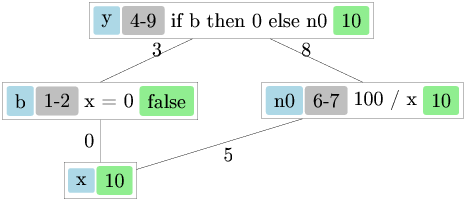First we evaluate `b` (attaching it as a dependent of `x` at time `0`) to get `false`. Then we evaluate `y` (attaching it as a dependent of `b` at time `3`): we check `b` and evaluate `n0` to get `10` (attaching it as a dependent of `x` at time `5`). Notice that we have a dependency edge from `y` to `n0`. This is not a true dependency, since we don’t recompute `y` when `n0` changes; rather the value of `y` is a proxy for `n0`, so when `n0` changes we just forward the new value to `y`.

What happens if we set `x = 20`? Both `b` and `n0` are stale since they depend on `x`. We re-run expressions in order of their start timestamp, so we run `b` and get `false`. Since the value of `b` has not changed, `y` is not stale. Then we re-run `n0`, so its value (and the value of `y` by proxy) becomes `5`.

What happens if we set `x = 0`? We run `b` and get `true`. Now `y` is also stale, and it is next in timestamp order. We first detach all the dependencies in the timestamp range `4`-`9` from the previous run of `y`: the dependency of `n0` on `x` and the proxy dependency of `y` on `n0`. This time we take the `then` branch, so we get `0` without attaching any new dependencies. We are done; no `Division_by_zero` exception.

Now we can see why it’s important to handle updates in timestamp order: the value which decides a control flow point (e.g. the test of an `if`) is always evaluated before the control branches (the `then` and `else` branches), so we have the chance to fix up the dependency graph before the branches are updated.

Garbage collection and cleanup functions

A node points to its dependencies (so it can read their values when computing its value), and its dependencies point back to the node (so they can mark it stale when they change). This creates a problem for garbage collection: a node which becomes garbage (from the point of view of the library user) is still attached to its dependencies, taking up memory, and causing extra recomputation.

The implementation of dynamic dependency graphs helps with this problem: as we have seen, when an expression is re-run, the dependencies attached in the course of the previous run are detached, including any dependencies for nodes which have become garbage. Still, until the expression that created them is re-run, garbage nodes remain attached.

Some other FRP implementations use weak pointers to store a node’s dependents, to avoid hanging on to garbage nodes. Since `froc` is designed to work in browsers (using ocamljs), weak pointers aren’t an option because they aren’t supported in Javascript. But even in regular OCaml, there are reasons to eschew the use of weak pointers:

First, it’s useful to be able to set up changeable expressions which are used for their effect (say, updating the GUI) rather than their value; to do this with a system using weak pointers, you have to stash the expression somewhere so it won’t be GC’d. This is similar to the problem of GCing threads; it doesn’t make sense if the threads can have an effect.

Second, there are other resources which may need to be cleaned up in reaction to changes (say, GUI event handler registrations); weak pointers are no help here. `Froc` gives you a way to set cleanup functions during a computation, which are run when the computation is re-run, so you can clean up other resources.

With `froc` there are two options to be sure you don’t leak memory: you can call `init` to clean up the entire system, or you can use `bind` to control the lifetime of changeables: for instance, you could have a changeable `c` representing a counter, do a computation in the scope of a bind of `c` (you can just ignore the value), then increment the counter to clear out the previous computation.

In fact, there are situations where `froc` cleans up too quickly—when you want to hang on to a changeable after the expression that attached it is re-run. We’ll see shortly how to avoid this.

Memoizing the previous run

Here is the `List.map` function, translated to work over lists where the tail is changeable.

``````  type 'a lst = Nil | Cons of 'a * 'a lst t

let rec map f lst =
lst >>= function
| Nil -> return Nil
| Cons (h, t) ->
let t = map f t in
return (Cons (f h, t))
``````

What happens if we run

``````  map (fun x -> x + 1) [ 1; 2; 3 ]
``````

? (I’m abusing the list syntax here to mean a changeable list with these elements.) Let’s see if we can fit the dependency graph on the page (abbreviating `Cons` and `Nil` and writing just `f` for the `function` expression):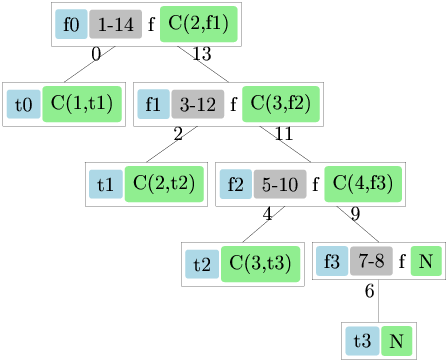(The dependency edges on the right-hand side don’t mean that e.g. `f0` depends directly on `f1`, but rather that the value returned by `f0``Cons(2,f1)`—depends on `f1`. We don’t re-run `f0` when `f1` changes, or even update its value by proxy as we did in the previous section. But if `f1` is stale it must be updated before we can consider `f0` up-to-date.)

Notice how the timestamp ranges for the `function` expressions are nested each within the previous one. There is a control dependency at each recursive call: whether we make a deeper call depends on whether the argument list is `Nil`.

So if we change `t3`, just `f3` is stale. But if we change `t0`, we must re-run `f0`, `f1`, `f2`, and `f3`—that is, the whole computation—detaching all the dependencies, then reattaching them. This is kind of annoying; we do a lot of pointless work since nothing after the first element has changed.

If only some prefix of the list has changed, we’d like to be able to reuse the work we did in the previous run for the unchanged suffix. `Froc` addresses this need with memo functions. In a way similar to ordinary memoization, a memo function records a table of arguments and values when you call it. But in `froc` we only reuse values from the previous run, and only those from the timestamp range we’re re-running. We can define `map` as a memo function:

``````  let map f lst =
let memo = memo () in
let rec map lst =
lst >>= function
| Nil -> return Nil
| Cons (h, t) ->
let t = memo map t in
return (Cons (f h, t)) in
memo map lst
``````

Here the `memo` call makes a new memo table. In the initial run we add a memo entry associating each list node (`t0`, `t1`, …) with its `map` (`f0`, `f1`, …). Now, suppose we change `t0`: `f0` is stale, so we update it. When we go to compute `map f t1` we get a memo hit returning `f1` (the computation of `f1` is contained in the timestamp range of `f0`, so it is a candidate for memo matching). `F1` is up-to-date so we return it as the value of `map f t1`.

There is a further wrinkle: suppose we change both `t0` and `t2`, leaving `t1` unchanged. As before, we get a memo hit on `t1` returning `f1`, but since `f2` is stale, so is `f1`. We must run the update queue until `f1` is up-to-date before we return it as the value of `map f t1`. Recall that we detach the dependencies of the computation we’re re-running; in order to update `f1` we just leave it attached to its dependencies and run the queue until the end of its timestamp range.

In general, there can be a complicated pattern of changed and unchanged data—we could change every other element in the list, for instance—so memoization and the update loop call one another recursively. From the timestamp point of view, however, we can think of it as a linear scan through time, alternating between updating stale computations and reusing ones which have not changed.

The memo function mechanism provides a way to keep changeables attached even after the expression that attached them is re-run. We just need to attach them from within a memo function, then look them up again on the next run, so they’re left attached to their dependencies. The quickhull example (source) demonstrates how this works.

Functional reactive programming and the event queue

Functional reactive programming works with two related types: behaviors are values that can change over time, but are defined at all times; events are defined only at particular instants in time, possibly (but not necessarily) with a different value at each instant. (Signals are events or behaviors when we don’t care which one.)

Events can be used to represent external events entering the system (like GUI clicks or keystrokes), and can also represent occurrences within the system, such as a collision between two moving objects. It is natural for events to be defined in terms of behaviors and vice versa. (In fact they can be directly interdefined with the `hold` and `changes` functions.)

In `froc`, behaviors are just another name for changeables. Events are implemented on top of changeables: they are just changeables with transient values. An incoming event sets the value of its underlying changeable; after changes have propagated through the dependency graph, the values of all the changeables which underlie events are removed (so they can be garbage collected).

Signals may be defined (mutually) recursively. For example, in the bounce example (source), the position of the ball is a behavior defined in terms of its velocity, which is a behavior defined in terms of events indicating collisions with the walls and paddle, which are defined in terms of the ball’s position.

`Froc` provides the `fix_b` and `fix_e` functions to define signals recursively. The definition of a signal can’t refer directly to its own current value, since it hasn’t been determined yet; instead it sees its value from the previous update cycle. When a recursively-defined signal produces a value, an event is queued to be processed in the next update cycle, so the signal can be updated based on its new current value. (If the signal doesn’t converge somehow this process loops.)

Related systems

`Froc` is closely related to a few other FRP systems which are change-driven and written in an imperative, call-by-value language:

FrTime is an FRP system for PLT Scheme. FrTime has a dependency graph and update queue mechanism similar to `froc`, but sorts stale nodes in dependency (“topological”) rather than timestamp order. There is a separate mechanism for handling control dependencies, using a dynamic scoping feature specific to PLT Scheme (“parameters”) to track dependencies attached in the course of evaluating an expression; in addition FrTime uses weak pointers to collect garbage nodes. There is no equivalent of `froc`’s memo functions. Reactivity in FrTime is implicit: you give an ordinary Scheme program, and the compiler turns each subexpression into a changeable value. There is no programmer control over the granularity of recomputation, but there is a compiler optimization (“lowering”) which recovers some performance by coalescing changeables.

Flapjax is a descendent of FrTime for Javascript. It implements the same dependency-ordered queue as FrTime, but there is no mechanism for control dependencies, and there are no weak pointers (since there are none in Javascript), so it is fairly easy to create memory leaks (although there is a special reference-counting mechanism in certain cases). Flapjax can be used as a library; it also has a compiler similar to FrTime’s, but since it doesn’t handle control dependencies, the semantics of compiled programs are not preserved (e.g. you can observe exceptions that don’t occur in the original program).

React is a library for OCaml, also based on a dependency-ordered queue, using weak pointers, without a mechanism for control dependencies.

Colophon

I used Mlpost to generate the dependency graph diagrams. It is very nice!

1.shouldn't the return of map be: return (Cons (f h, t)) instead of: return (Cons (h, t)) [the same goes for memoized version] ?

2.Oops, of course, thanks for the correction.

3.Hello Jake,

Thanks for your excellent froc library, i had given up learning frp until I came across your blog entry and library.

1)
In the example, the definition of y should be :
let y = bind2 b n0 (fun b _ -> if b then return 0 else n0)

2)
I am baffled by the following code :

let print_nl s = print_string s; print_newline()

let u,send_u = make_cell 1
let v,send_v = make_cell 1
let x = blift2 u v (fun u v -> u/v)
let y = blift2 u v (fun u v -> u*v)

let x' = blift x (fun _ -> print_nl "x changed")
let y' = blift y (fun _ -> print_nl "y changed")

Now in the toplevel :
send_v 2;;
x changed
y changed
- : unit = ()

so far so good, but when I set v to 0 :

send_v 0;;
y changed
- : unit = ()

Does froc "holds and hides" the Division_by_zero exception and only exposes it only when I do "Froc.sample x" ?
If so, why isn't the x' notifier never get triggered for that value, even when I do an explicit sample ?

2bis)
In the example above, is there any way to "unbind" the x' behavior so that any subsequent update of x wont trigger the print_nl "callback"
I guess the cleanup function is what I need, it would be great if you could provide me an example.

3)
In the ddg section, you explain the timestamp update mechanism. Yet, if the "b" node had an initial timestamp value of 1 and "n0" a ts value of 0, this wouldnt work.

Does Frog somehow (seems impossible to me since it would require statical code analysis) figures out that the "b node" determines the control flow and hence gets allocated a lesser timestamp than the n0 node ?
Or is it simply because we defined the b behavior before n0 ? (it dont think so because i switched the order and didnt observe a different result)
Or because the same phenomenon as in 2) occurs ?

4)
Is there any controlled way to fire n events simultaneously
(so that everything gets treated within the same update cycle ) ?

let's consider the behavior u = f(v,w,x,y,z) above. I want to change
"atomically" (within the same update cycle) the 4 inputs without trigering 4 distinct update cycles.

Thanks in advance and sorry for this long comment

4.Thanks for the comment, I'm glad you are enjoying froc.

1) You are right, thanks for the fix.

2) Only successful values are propagated via bind, not exceptions. If you want to attach a notifier which receives exceptions you can use notify_result_b, which for an 'a behavior passes an 'a result (i.e. Value of 'a | Fail of exn) to the notification function. You could also use try_bind, catch, etc. to handle exceptions.

2bis) For notifications set with notify_b_cancel you are returned a cancellation handle; call Froc.cancel on it to cancel the notification. Other kinds of attachment (bind, notify_b, etc.) are cancelled automatically when their enclosing scope is cleaned up.

3) Timestamps are allocated in order as evaluation proceeds; calls to bind force an evaluation order. So in the second example, 100/x is bound to x within the bind of b, so its node has a later timestamp.

4) One way to accomplish it is to fire a single event consisting of the tuple of values you want, then take the tuple apart in an event handler and pass the parts where you need them.

5.Hello! Noting that Froc updates are 2 years old, is Froc dormant, or do you use it and it simply is a completed product without need of fixes or extensions? I've written about Froc and implemented a Flows module modeled after "Deprecating the Observer Pattern with Scala.React" paper, in lecture 10 at http://www.ii.uni.wroc.pl/~lukstafi/pmwiki/index.php?n=Functional.Functional#curlecture

6.Cool, nice to see someone using it. Unfortunately I don't do OCaml for work any more and I have no opportunity to use or improve froc at the moment, so it is dormant.

I think froc is usable as it stands (although I have not tried building it with a recent OCaml), but there is certainly more that could be done with it. One direction I'd like to go is support for "traceable" data structures as in

http://www.umut-acar.org/publications/pldi2010.pdf

or some other efficient way to handle small changes to large data structures.

7.FROC is now available in OPAM via `opam install froc`

8.re building it -- fwiw i've recently packaged Froc up for opam, and it's now in the main repo. seemed to build just fine using ocaml 4.00.1 and 4.01.0dev+trunk, modulo the Makefile patches i had to include in the opam package. thought you might be interested...